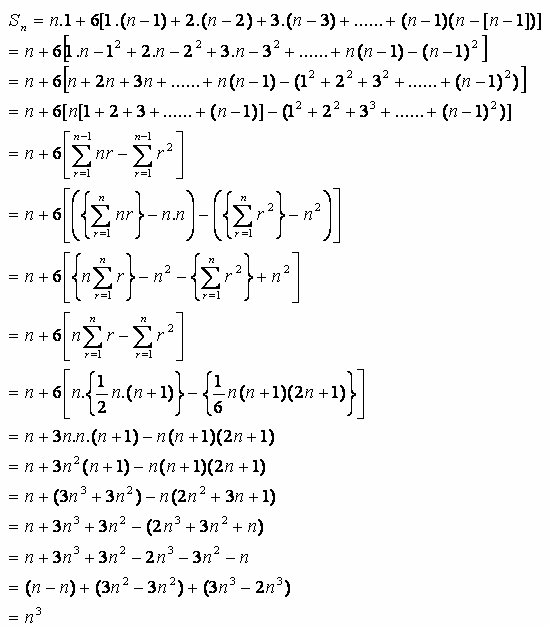Cubes and Cube Roots

Calculating the cube root of integer perfect cubes

For perfect cubes, it is possible to calculate the root by hand.

For n3, where n is an integer, the method of extracting the root is as follows.

 A B Root Comments a n0=n3 Place n3under Root, call this n0 b 1 n1 = n0 - 1 Place 1 under A and subtract from Root, new Root is now n1 c 1 Place 1 under Root_Number_B d1 2 3 Add 1 to A, add to last B value e1 4 7 n2 = n1 - 7 Add 2 to A, add to last B value, now subtract new B from Root, new root is now n2 d1 5 12 Add 1 to A, add to last B value e1 7 19 n3 = n2 - 19 Add 2 to A, add to last B value, now subtract new B from Root, new root is now n3

Repeat the process d and e until root n = 0. The value of the Root_Number e when n = 0 is then added to 2 and the sum is divided by 3 which should give the cube root of n^3.

A real example follows.

4x4x4 = 64, so the cube root of 64 is 4.

 A B Root 64 1 63 1 2 3 4 7 56 5 12 7 19 37 8 27 10 37 0

The value of A when Root = 0 is 10. Adding 2 to 10 and dividing by 3 = 12/3 = 4

Series

The numbers which are subtracted from the Root form a series

Sn = 1 + 7 + 19 + 37 + .....

If one looks at the sum, the number of terms relates to the cube root of the sum itself.

13 term 1 = 1
23 term 8 = 1 + 7
33 term 27 = 1 + 7 + 19
43 term 64 = 1 + 7 + 19 + 37
.
.
n3 term = 1 + 7 + 19 + 37 + …… + (un)

The expression for n3 sum can be in the form

Where

(1st term) 1 = 1
(2nd term) 7 = 1 + 6
(3rd term) 19 = 1 + 6 + 12
(4th term) 37 = 1 + 6 + 12 + 18
.
.

By inspection, we see that each successive term contains 1 + a number of terms values which are multiples of 6. Since the first term is 1, the last term is the 6.(n-1).

(nth term) = 1 + 6 + 12 + 18 + 24 + … … + 6.(n-1)

Inspecting the terms, we find that

• 1 occurs n times
• 6 = 6.1 occurs n-1 times
• 12 = 6.2 occurs n-2 times
• 18 = 6.3 occurs n-3 times
• 24 = 6.4 occurs n-4 times

The term 6.(n-1) will appear n-(n-1) times or just once.

Thus the sum becomesThe mathematical summations indicated by the sigma signs are standard identities [Tuckey & Armistead, p.211]. So, from the generalised equation of the summation for

Sn = 1 + 7 + 19 + 37 + ...... + un

and identified a

we have found it to be equivalent to the cube of n.

That is,

Sn = n + 6[ (n-1) + 2(n-2) + 3(n-3) + ... + (n-1)(n-[n-1]) ] = n3

Reference

Algebra. C.O. Tuckey & W. Armistead. Longmans, Green and Co., 1958